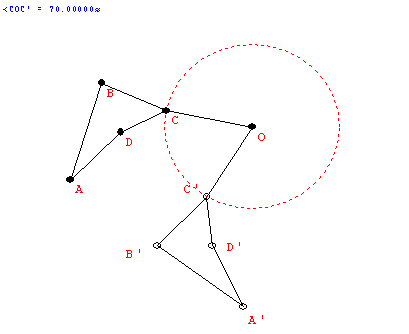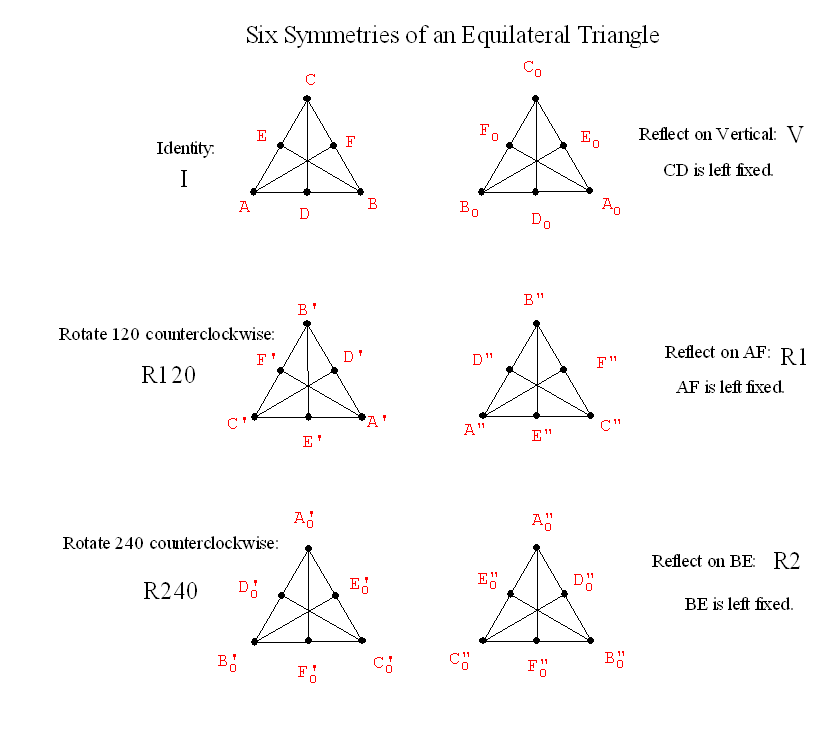September 18
Discuss Project proposal... Some suggestions for topics.... Form partnerships!!!!

Review from last class:Symmetry Ideas
• Reflective symmetry: BI LATERAL SYmMETRY
• Folding line: "axis of symmetry"
• The "flip."
• The "mirror."
•• R(P) = P'  : A Transformation. Before: P .... After : P'
• If P is on the line (axis), then F(P)=P. "P remains fixed by the reflection."
• If P is not on the axis, then the line PP' is perpendicular to the axis and if Q is the point of intersection of PP' with the axis then m(PQ) = m(p'Q).

• Definition: We say F has a reflective symmetry wrt a line l if  there is a reflection  R about the line l where  R(P)=P' is still an element of F for every P in F....i.e.. R (F) = F. l is called the axis of symmetry.
• Examples of reflective symmetry:

• Rotational Symmetry.
• Center of rotation. "rotational pole" (usually O) and angle/direction of rotation.
• The "spin."
•• R(P) = P' : A transformation
• If O is the center then F(O) = O.
• If the angle is 360 then F(P) = P for all P.... called the identity transformation.
• If  the angle is between 0 and 360 then only the center remains fixed.
• For any point P the angle POP'  is the same.
• Examples of rotational symmetry.

• Show Video on symmetry from For All Practical Purposes.

•  Now what about finding all the reflective and rotational symmetries of a single figure?

•• Why are there only six?

Before: A                              After : A  or    B  or     C

Suppose I know where A goes:

What about B?  If A -> A     Before: B   After: B   or C

If  A ->B        Before:B     After: A or C

If  A ->C       Before: B       After A or B

By an analysis of a "tree" we count there are exactly and only 6 possibilities for where the vertices can be transformed.

• What about combining transformations to give new symmetries:

Think of a symmetry as a transformation, for example, F will mean reflection across the line that is the vertical altitude of the equilateral triangle.

Then let's consider a second symmetry, called R, which will rotate the equilateral triangle counterclockwise about its center O by 120 degrees.

We now can think of first performing F to the figure and then performing R to the figure.
We will denote this F*R... meaning F followed by R.
[Note that order can make a difference here, and there is an alternative  convention for this notation that would reverse the order and say that R*F means F followed byR.]
Does the resulting transformation also leave the equilateral  covering the same position in which it started?

F*R120 = V*R120 = R2

If so it is also a symmetry.... which of the six is it?

What about  S*R? This gives a  "product" for symmetries.
If S and R are any symmetries of a figure then S*R is also a symmetry of the figure.

A "multiplication" table for Symmetries:
 * Id R120 R240 F G H Id I R120 R240 R1 R240 I F=V R2 I R120 G=R1 I H=R2 I
Do Activity. This shows that R120*F = R1

Called this the Group of symmetries of the equilateral triangle.

Given any figure we can talk about the group of its symmetries.
Does a figure always have at least one symmetry? Yes... The Identity symmetry.
Such a symmetry is called the trival symmetry.

So we can compare objects for symmetries....
how many?
does the multiplication table for the symmetries look the same in some sense?

What about the symmetries of a Frieze Pattern on a Strip....

Translations and Glide Reflections  [180 degree Rotations and Reflections]

...|p|q|p|q|p|q|p|q|p|q|p|...

This pattern has vertical axes for reflective symmetries between each p and q... and a translation symmetry taking each letter to the next letter of the ssame type... and also twice as far, and three times as far , and more....

The same is true for the following pattern:

...|d|b|d|b|d|b|d|b|d|b|d|...

The following pattern also has glide reflection symmetry, for example taking p to b and q to d, etc.
It also has a 180 degree rotational symmetry with center midway between the vertical reflection axes and the letter p and d or q and b.

...|p|q|p|q|p|q|p|q|p|q|p|...
...|d|b|d|b|d|b|d|b|d|b|d|...

Next time: more on symmetries of frieze patterns ....

What about the symmetries of a tiling?